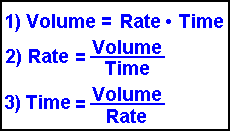Flow Rate Ultra Calculator Solves for Volume Time or Rate Scroll to the bottom for instructions

Do you want to solve for:
Volume     Time   or   Rate ?

 VOLUME *** V O L U M E ************** Cubic Centimeters Cubic Feet Cubic Inches Cubic Meters Cubic Millimeters Cubic Yards gallons (U.S.) liters
 TIME *** T I M E ********************* Seconds Minutes Hours
 RATE *** R A T E *********************** cubic centimeters / second cubic centimeters / minute cubic centimeters / hours cubic feet / second cubic feet / minute cubic feet / hour cubic inches / second cubic inches / minute cubic inches / hour cubic meters / second cubic meters / minute cubic meters / hour cubic millimeters / second cubic millimeters / minute cubic millimeters / hour cubic yards / second cubic yards / minute cubic yards / hour gallons (U.S.) / second gallons (U.S.) / minute gallons (U.S.) / hour liters / second liters / minute liters / hour

 I N S T R U C T I O N SThe above 3 formulas are used for solving problems involving flow rate calculations. If you know 2 of the 3 variables the third can be calculated. As usual, here at www.1728.com, we have a calculator that will do all the work for you. This ultra calculator is special by allowing you to choose among a great variety of units. Unlike other calculators, you are NOT confined to inputting volume in liters, time in minutes, etc. making this calculator quite versatile. Let's see some examples. 1) Using a garden hose that has a rate of .75 gallons per minute, it took you 2.5 hours to fill up a swimming pool. What is the volume of the pool? The most important step in using this calculator is FIRST CHOOSE WHAT YOU ARE SOLVING FOR. In this case we are solving for VOLUME so click on that button. Enter 2.5 in the "time" box and choose hours from its menu. Enter .75 in the "rate" box and choose gallons per minute from its menu. Click the CALCULATE button and you will see this equals 15.039 cubic feet PLUS you get to see the answer in 7 other different units !! 2) A 9 cubic yard cement truck is pouring cement at a rate of 80 cubic feet per minute. How long does it take to pour out all the cement? FIRST CHOOSE WHAT YOU ARE SOLVING FOR. In this case we are solving for TIME so click on that button. Enter 9 in the "volume" box and choose cubic yards from the menu. Enter 80 in the "rate" box and choose cubic feet per minute from the menu. Click the CALCULATE button and your answer will be 182.25 seconds OR 3.0375 minutes OR 0.050625 hours. 3) A faucet can fill a 1 gallon jug in 90 seconds. What is the flow rate? FIRST CHOOSE WHAT YOU ARE SOLVING FOR. In this case we are solving for RATE so click on that button. Enter 1 in the "volume" box and choose gallons from the menu. Enter 1.5 in the "time" box and choose minutes from its menu. Click the CALCULATE button and your answer is 42.06 cubic centimeters per second PLUS answers in 23 other units. Here's hoping this calculator helps you with those math problems. Significant Figures >>> For easy readability, the answers are displayed in a "significant figure" format so you will not see answers such as 77.3333333333333333. Numbers larger than 1,000 will be displayed in scientific notation and with the same number of significant figures specified. You may change the significant figures displayed by changing the number in the box above. Internet Explorer and most other browsers, will display the answers properly but there are a few browsers that will show no output whatsoever. If so, enter a zero in the box above. This eliminates all formatting but it is better than seeing no output at all.